Glottis

# Glottis

## Overview

glottis implements an analytical model that approximates a signal that the human glottis makes. It is based on the works of Liljencrants and Fant. Unlike the corresponding vocal tract that usually accompanies this vocla tract, the glottis is an anlytical model rather than physically based. This undelrying waveform is generated via a mathematical equation derived emprically rather than from physical principles.

The implementation used here is based off of the one found in Pink Trombone by Neil Thapen.

## Tangled Files

This tangles to two files: glottis.c and glottis.h. Defining SK_GLOTTIS will expose the contents in the struct.

<<glottis.c>>=
#include <math.h>
#include <stdlib.h>
#define SK_GLOTTIS_PRIV
#include "glottis.h"

#ifndef M_PI
#define M_PI 3.14159265358979323846
#endif

<<static_funcdefs>>
<<funcs>>

<<glottis.h>>=
#ifndef SK_GLOTTIS_H
#define SK_GLOTTIS_H

#ifndef SKFLT
#define SKFLT float
#endif

<<typedefs>>
<<funcdefs>>

#ifdef SK_GLOTTIS_PRIV
<<structs>>
#endif
#endif

## Struct and Initialization

The glottis is initialized with sk_glottis_init.

<<funcdefs>>=
void sk_glottis_init(sk_glottis *glot, SKFLT sr);

The struct is defined in a struct called sk_glottis.

<<typedefs>>=
typedef struct sk_glottis sk_glottis;

<<funcs>>=
void sk_glottis_init(sk_glottis *glot, SKFLT sr)
{
glot->freq = 140; /* 140Hz frequency by default */
glot->tenseness = 0.6; /* value between 0 and 1 */
glot->T = 1.0/sr; /* big T */
glot->time_in_waveform = 0;
setup_waveform(glot);
}

Contents of sk_glottis displayed below. This will eventually be elaborated more:

<<structs>>=
struct sk_glottis {
SKFLT freq;
SKFLT tenseness;
SKFLT Rd;
SKFLT waveform_length;
SKFLT time_in_waveform;

SKFLT alpha;
SKFLT E0;
SKFLT epsilon;
SKFLT shift;
SKFLT delta;
SKFLT Te;
SKFLT omega;

SKFLT T;
};

## Parameters

### Frequency

Set with sk_glottis_freq.

<<funcdefs>>=
void sk_glottis_freq(sk_glottis *glot, SKFLT freq);

<<funcs>>=
void sk_glottis_freq(sk_glottis *glot, SKFLT freq)
{
glot->freq = freq;
}

### Tenseness

Set with sk_glottis_tenseness

<<funcdefs>>=
void sk_glottis_tenseness(sk_glottis *glot, SKFLT tenseness);

<<funcs>>=
void sk_glottis_tenseness(sk_glottis *glot, SKFLT tenseness)
{
glot->tenseness = tenseness;
}

## Calculating the waveform

The glottis model works by mathematically generating a new waveform every time a new period begins. The period length is determined by the given frequency.

<<static_funcdefs>>=
static void setup_waveform(sk_glottis *glot);

<<funcs>>=
static void setup_waveform(sk_glottis *glot)
{
SKFLT Rd;
SKFLT Ra;
SKFLT Rk;
SKFLT Rg;

SKFLT Ta;
SKFLT Tp;
SKFLT Te;

SKFLT epsilon;
SKFLT shift;
SKFLT delta;
SKFLT rhs_integral;

SKFLT lower_integral;
SKFLT upper_integral;

SKFLT omega;
SKFLT s;
SKFLT y;
SKFLT z;

SKFLT alpha;
SKFLT E0;

<<calculate_waveform_length>>
<<calculate_rd>>
<<calculate_ra_rk>>
<<calculate_rg>>
<<calculate_timing_parameters>>
<<calculate_epsilon_shift_delta>>
<<calculate_integrals>>

omega = M_PI / Tp;
s = sin(omega * Te);

y = -M_PI * s * upper_integral / (Tp*2);
z = log(y);
alpha = z / (Tp/2 - Te);
E0 = -1 / (s * exp(alpha*Te));

<<update_variables_in_struct>>
}

To begin, both waveform_length and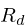(Rd) are calcuated.

The variable waveform_length is the period of the waveform based on the current frequency.

<<calculate_waveform_length>>=
glot->waveform_length = 1.0 / glot->freq;is part of a set of normalized timing parameters used to calculate the time coefficients described in the LF model, as described in a 1997 Fant paper.is derived from the tenseness parameter, and then clamped to be in between 0.5 and 2.7, as these are good approximations (as observed in a paper titled "Glottal Source Modelling for Singing Voice Synthesis" by Hui-Ling Lu and Julius O Smith in 2000).

<<calculate_rd>>=
glot->Rd = 3 * (1 - glot->tenseness);
Rd = glot->Rd;
if (Rd < 0.5) Rd = 0.5;
if (Rd > 2.7) Rd = 2.7;

The other timing parameters(Ra),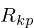(Rg), and(Rk) can be computed in terms of(Rd), which is why this gets computed first. The equations described below have been derived using linear regression: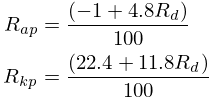In code, these have been worked out to shave off a division operation.

<<calculate_ra_rk>>=
Ra = -0.01 + 0.048*Rd;
Rk = 0.224 + 0.118*Rd;(Rg) is derived using the results fromandin the following equation described in the Fant 1997 paper: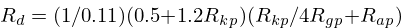Which yields: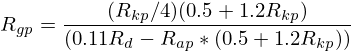<<calculate_rg>>=
Rg = (Rk/4)*(0.5 + 1.2*Rk)/(0.11*Rd-Ra*(0.5+1.2*Rk));

The parameters approximating R_a, R_g, and R_kcan be used to calculate the timing parameters T_a, T_p, and T_e in the LF model: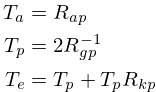<<calculate_timing_parameters>>=
Ta = Ra;
Tp = (SKFLT)1.0 / (2*Rg);
Te = Tp + Tp*Rk;

At this point, the timing variables can be used to calculate three more variables needed: epsilon, shift, and delta.

<<calculate_epsilon_shift_delta>>=
epsilon = (SKFLT)1.0 / Ta;
shift = exp(-epsilon * (1 - Te));
delta = 1 - shift;

These are then used to calculate the integrals (TODO: understand this a bit better).

<<calculate_integrals>>=
rhs_integral = (SKFLT)(1.0/epsilon) * (shift-1) + (1-Te)*shift;
rhs_integral = rhs_integral / delta;
lower_integral = - (Te - Tp) / 2 + rhs_integral;
upper_integral = -lower_integral;

More components here. The original Voc program didn't describe these in much detail, so...

Finally, update the variables in the struct.

<<update_variables_in_struct>>=
glot->alpha = alpha;
glot->E0 = E0;
glot->epsilon = epsilon;
glot->shift = shift;
glot->delta = delta;
glot->Te = Te;
glot->omega = omega;

## Computation

A single sample of audio is computed with sk_glottis_tick.

<<funcdefs>>=
SKFLT sk_glottis_tick(sk_glottis *glot);

<<funcs>>=
SKFLT sk_glottis_tick(sk_glottis *glot)
{
SKFLT out;
SKFLT aspiration;
SKFLT noise;
SKFLT t;

out = 0;

<<increment_waveform_timer>>
<<update_waveform_if_needed>>

<<compute_waveform>>
<<compute_aspiration_noise>>

return out;
}

The waveform timer is incremented. This measures the duration in the period.

<<increment_waveform_timer>>=
glot->time_in_waveform += glot->T;

If the waveform reaches the end of the period, update the parameters for the next period.

<<update_waveform_if_needed>>=
if (glot->time_in_waveform > glot->waveform_length) {
glot->time_in_waveform -= glot->waveform_length;
setup_waveform(glot);

}

Compute the glottal waveform component. This varies depending on where it is in the period. This presumably comes from the LF model, but I'll need to check the papers again because I forget.

<<compute_waveform>>=
t = (glot->time_in_waveform / glot->waveform_length);

if (t > glot->Te) {
out = (-exp(-glot->epsilon * (t-glot->Te)) + glot->shift) / glot->delta;
} else {
out = glot->E0 * exp(glot->alpha * t) * sin(glot->omega * t);
}

Aspiration noise is approximated using white noise, and mixed into the glottal signal. How much is mixed in is inversely proportionally to the tenseness amount.

At the time of writing, the built-in rand function is used to produce the noise. It works well enough, but varies from platform to platform slightly. The hope is to later build an internal random number generator that is more consistent and portable.

<<compute_aspiration_noise>>=
/* TODO: build more portable RNG */
noise = 2.0 * ((SKFLT) rand() / RAND_MAX) - 1;
aspiration = (1 - sqrt(glot->tenseness)) * 0.3 * noise;
aspiration *= 0.2;
out += aspiration;